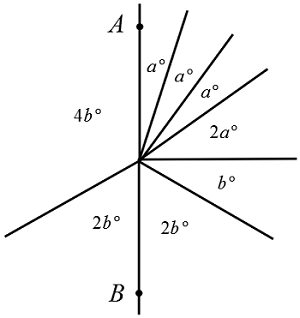# SAT AnglesIn the figure above, segment $\overline{AB}$ is a straight line. What is the value of $a^\circ + b^\circ ?$

(A) $\ \ 12$
(B) $\ \ 14$
(C) $\ \ 30$
(D) $\ \ 48$
(E) $\ \ 50$

×